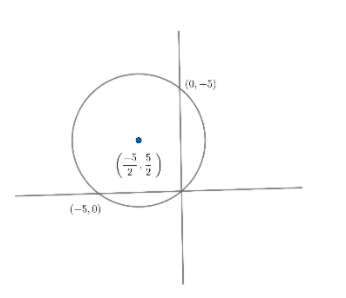Courses
Courses for Kids
Free study material
Free LIVE classes
More# Assertion (A): Tangents cannot be drawn from the point $\left( 1,\lambda \right)$ to the circle ${{x}^{2}}+{{y}^{2}}+5x-5y=0$, if $2<\lambda <3$.Reason (R): ${{\left( 1+\dfrac{5}{2} \right)}^{2}}+{{\left( \lambda -\dfrac{5}{2} \right)}^{2}}<{{\left( \dfrac{5}{2} \right)}^{2}}+{{\left( \dfrac{5}{2} \right)}^{2}}$ (A) Both A and R are individually true and R is the correct explanation of A. (B) Both A and R are individually true and R is not the correct explanation of A. (C) A is true but R is false.(D) A is false but R is true.(a) A(b) B(c) C(d) D

Last updated date: 16th Mar 2023
Total views: 306.9k
Views today: 5.86kVerified
306.9k+ views
Hint: If a point lies outside the circle, we can draw two tangents from that point to the circle and if the point lies inside the circle, we cannot draw any tangent from that point to the circle.

In the question, a circle ${{x}^{2}}+{{y}^{2}}+5x-5y=0$ has been given.
A point $\left( 1,\lambda \right)$ from which tangents are to be drawn to the circle is also given in the question.
The general equation of a circle is given by ${{x}^{2}}+{{y}^{2}}+2gx+2fy+C=0$. The centre of which is given by $\left( -g,-f \right)$ and the radius is $=\sqrt{{{g}^{2}}+{{f}^{2}}-C}$.
So for the given circle ${{x}^{2}}+{{y}^{2}}+5x-5y=0$, we can observe that $g=\dfrac{5}{2}$, $f=-\dfrac{5}{2}$ and $C=0$. Substituting these values of $g,f$ and $C$ in the formula for centre and radius, we get
Centre $=\left( -\dfrac{5}{2},\dfrac{5}{2} \right)$
Radius $r=\sqrt{{{\left( \dfrac{5}{2} \right)}^{2}}+{{\left( -\dfrac{5}{2} \right)}^{2}}}$
$r=\dfrac{5}{2}\sqrt{1+1}$
$\Rightarrow r=\dfrac{5}{2}\sqrt{2}$
From the results obtained, we can draw the circle as shown below.To draw a tangent from a particular point to the circle, we know that the point must be outside the circle. This means that the distance between the point and the centre of the circle must be greater than the radius of the circle.
We know that the distance formula to find the distance between any two points with coordinates $\left( x,y \right)$ and $\left( x',y' \right)$ is given by
$d=\sqrt{{{(x-{x}')}^{2}}+{{(y-{y}')}^{2}}}$
Therefore, the distance between point $\left( 1,\lambda \right)$ and centre $\left( -\dfrac{5}{2},\dfrac{5}{2} \right)$ can be calculated using the distance formula as:
$d=\sqrt{{{\left( 1-\left( -\dfrac{5}{2} \right) \right)}^{2}}+{{\left( \lambda -\dfrac{5}{2} \right)}^{2}}}$
$d=\sqrt{{{\left( 1+\dfrac{5}{2} \right)}^{2}}+{{\left( \lambda -\dfrac{5}{2} \right)}^{2}}}.............\left( i \right)$
We know that the radius of the circle is given by $r=\sqrt{{{g}^{2}}+{{f}^{2}}-C}$ for the general circle ${{x}^{2}}+{{y}^{2}}+2gx+2fy+C=0$. So, for the circle, ${{x}^{2}}+{{y}^{2}}+5x-5y=0$ we have the radius as
$r=\sqrt{{{\left( \dfrac{5}{2} \right)}^{2}}+{{\left( -\dfrac{5}{2} \right)}^{2}}}.........\left( ii \right)$
Like we discussed in the above paragraph, for a tangent to be drawn from $\left( 1,\lambda \right)$, the distance $\left( d \right)$ between the point $\left( 1,\lambda \right)$ and the centre of the circle $\left( -\dfrac{5}{2},\dfrac{5}{2} \right)$ must be greater than the radius of the circle, i.e.
$d>r$or ${{d}^{2}}>{{r}^{2}}$
From $\left( i \right)$ and $\left( ii \right)$, substituting the values of $d$ and $r$, we get
${{\left( 1+\dfrac{5}{2} \right)}^{2}}+{{\left( \lambda -\dfrac{5}{2} \right)}^{2}}>{{\left( \dfrac{5}{2} \right)}^{2}}+{{\left( \dfrac{5}{2} \right)}^{2}}$
If we want no tangent to be drawn from this point, the distance $\left( d \right)$ between the point $\left( 1,\lambda \right)$ and the centre of the circle $\left( -\dfrac{5}{2},\dfrac{5}{2} \right)$ must be less than the radius of the circle. This means that
$dFrom \left( i \right) and \left( ii \right), substituting the values of d and r, we get \[{{\left( 1+\dfrac{5}{2} \right)}^{2}}+{{\left( \lambda -\dfrac{5}{2} \right)}^{2}}<{{\left( \dfrac{5}{2} \right)}^{2}}+{{\left( \dfrac{5}{2} \right)}^{2}}..............\left( iii \right)$
Solving this inequality, we get
${{\left( \dfrac{7}{2} \right)}^{2}}+{{\left( \lambda -\dfrac{5}{2} \right)}^{2}}<2{{\left( \dfrac{5}{2} \right)}^{2}}$
$\Rightarrow \dfrac{49}{4}+{{\lambda }^{2}}+\dfrac{25}{4}-5\lambda <2\times \dfrac{25}{4}$
$\Rightarrow \dfrac{49}{4}+{{\lambda }^{2}}+\dfrac{25}{4}-5\lambda <\dfrac{50}{4}$
$\Rightarrow {{\lambda }^{2}}-5\lambda +\dfrac{49+25-50}{4}<0$
$\Rightarrow {{\lambda }^{2}}-5\lambda +\dfrac{24}{4}<0$
$\Rightarrow {{\lambda }^{2}}-5\lambda +6<0$
$\Rightarrow {{\lambda }^{2}}-2\lambda -3\lambda +6<0$
$\Rightarrow \lambda \left( \lambda -2 \right)-3\left( \lambda -2 \right)<0$
$\Rightarrow \left( \lambda -3 \right)\left( \lambda -2 \right)<0$
$\Rightarrow \lambda \in \left( 2,3 \right)$
Or $2<\lambda <3............(iv)$
Hence from inequation $\left( iii \right)$ and inequation $\left( iv \right)$, both A and R are individually true and from (iii) R is the correct explanation of A.

Option (a) is the correct answer

Note: There is an alternate method to check if a point lies inside the circle or outside the circle. We just have to substitute the point in the equation of the circle. If the value obtained is negative, the point lies inside and if it is positive, it lies outside.